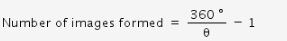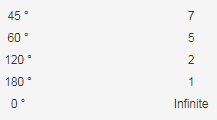# How many images are formed when two plane mirrors are set at an angle

How many images are formed when two plane mirrors are set at an angle of 45 degrees, 60 degrees, 120 degrees, and 180 degrees? please give answer with proper explanation.
How many images will be formed when mirrors are at parallel?

The formula to find the number of images (n) formed between two plane mirrors inclined at angle,θ between them is as follows:Substituting the different values of angles between two inclined mirrors we can tabulate the answer as follows:
Angle between the two mirrors Number of images formed (θ)